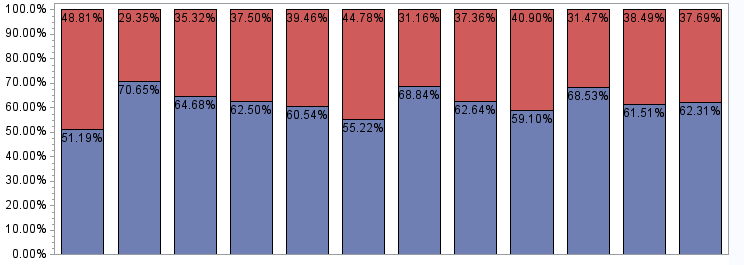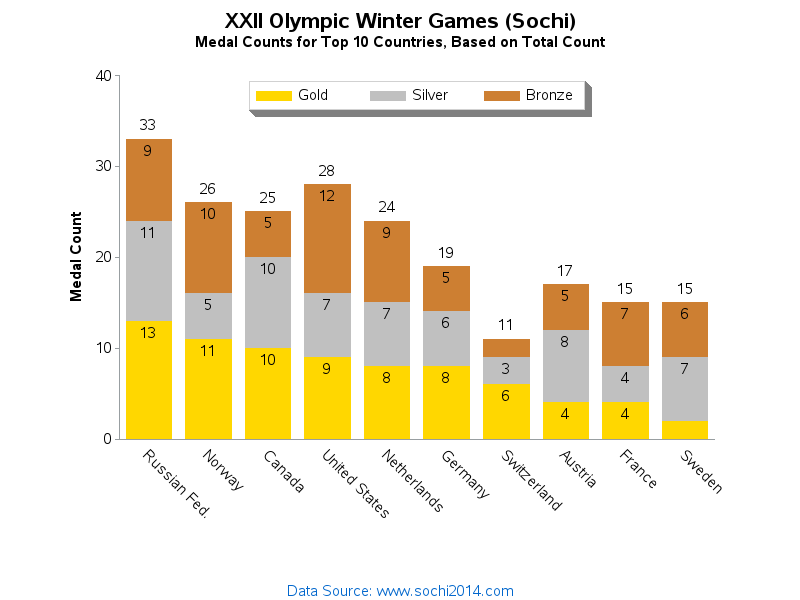## Ascending Order of my Graph

Hi,

I plotted a chart which is attached. But I'd like to know is there any way that I can plot in an ascending order of the BLUE portion of the graph. I want my chart to be in ascending order (blue color).

Help me.

Naresh3 REPLIES 3DanH_sas
SAS Super FREQ

## Re: Ascending Order of my Graph

Is the graph part of the code available for posting? And, what version of SAS are you using?

## Re: Ascending Order of my Graph

proc gchart data=Multiplot;

vbar Client / sumvar = percent discrete inside = subpct

subgroup = cross;

run;

quit;

9.3 Version

## Re: Ascending Order of my Graph

Here is an example where I sort the bars by 1 of the colored bar segments (in this case, the number of gold Olympic medals)...

I sort the data in the desired order, and then assign a 'bar_order' variable, and plot the bars by those numeric bar_order values.  I then create a user-defined-format so the numeric bar-order values show up on the axis as the desired text (rather than their numeric value).Discussion stats
• 3 replies
• 925 views
• 0 likes
• 3 in conversation